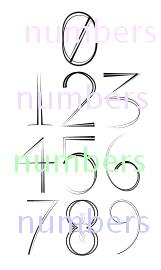## Friday, 5 October 2012

### Numbers Quiz (CTET/Class6/Class7/Class 8)

Numbers Quiz
(CTET/Class6/Class7/Class 8)

Q1: 2, 4, 6, 8, 10 ... are

(a) prime numbers
(b) even numbers
(c) odd numbers
(d) none of these

Q2: The roman number CD in decimal form is

(a) 100
(b) 500
(c) 400
(d) 0

Q3: Total number of prime numbers up to 100 are:

(a) 20
(b) 24
(c) 25
(d) 28

Q4:  The only even number which is prime

(a) 2
(b) 0
(c) 0 and 2
(d) none

Q5: If 'p' is an odd number, 'q' is an even number and 'r' is an odd number. Then p+q+r is

(a) odd number
(b) even number
(c) any non-prime number
(d) always a prime number

Q6: The two numbers which have  only '1' as the common factor are called

(a) prime numbers
(b) composite numbers
(c) primary numbers
(d) co-prime numbers

Q7: The two consecutive natural numbers will always be

(a) odd numbers
(b) composite numbers
(c) co-primes
(d) even numbers

Q8: The smallest number of four digits is:

(a) 0001
(b) 0010
(c) 1000
(d) 1001

Q9: The product of two prime numbers is

(a) composite number
(b) prime number
(c) odd number
(d) even number

Q10: In the series 13,16,49,169, 256, ... what shall be the next number

(a) 512
(b) 169
(c) 361
(d) 289

Q11: Sum of of first 'n' even numbers is:

(a) n×(n+1)/2
(b) n×(n+1)
(c) n2
(d) n2+1

1: (b) even numbers
2: (c) 400
3: (c) 25
4: (a) 2
5: (b) even number
6: (d)co-prime numbers
7: (c) co-primes
8: (c) 1000
9: (a) composite number
10: (b) 169
11: (b) n×(n+1)